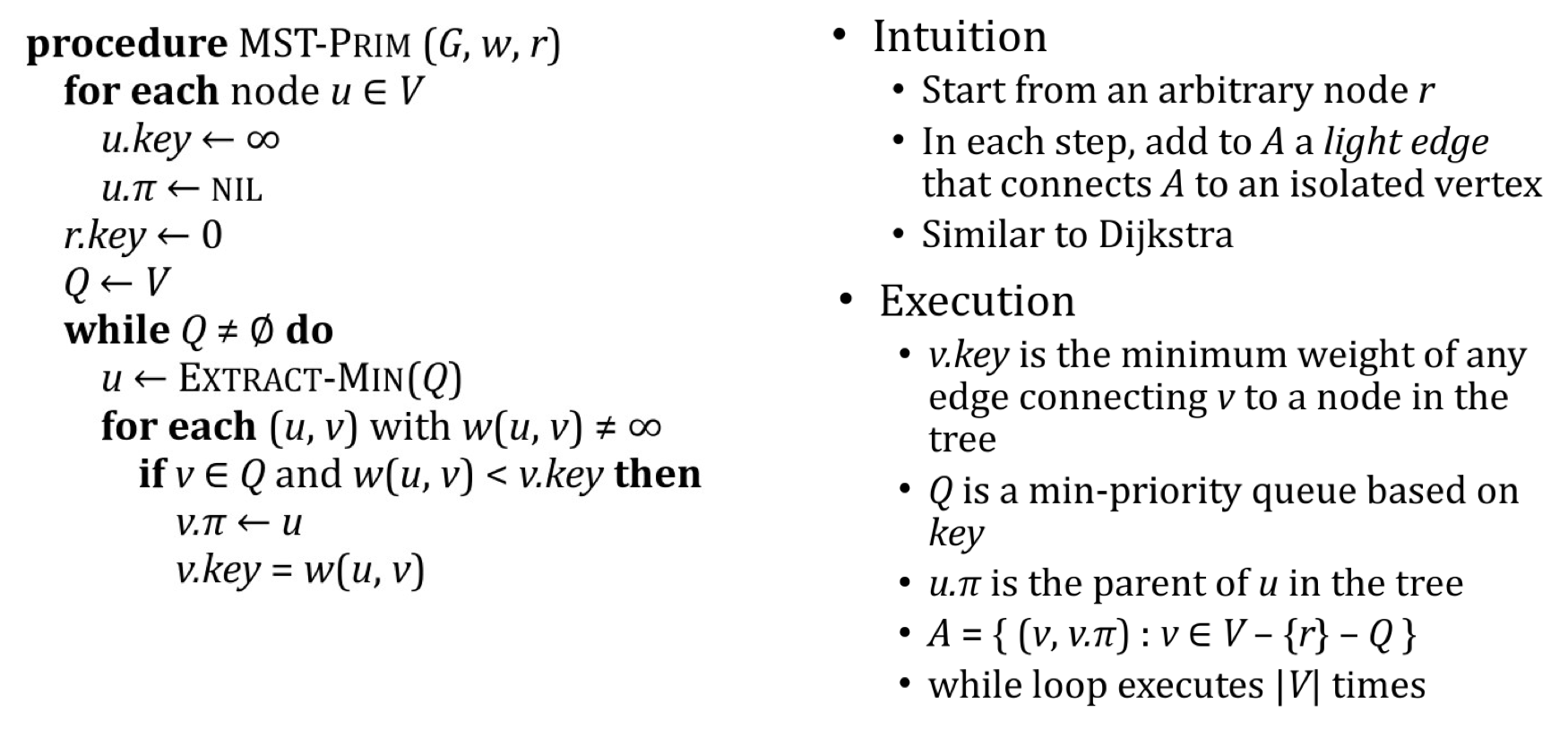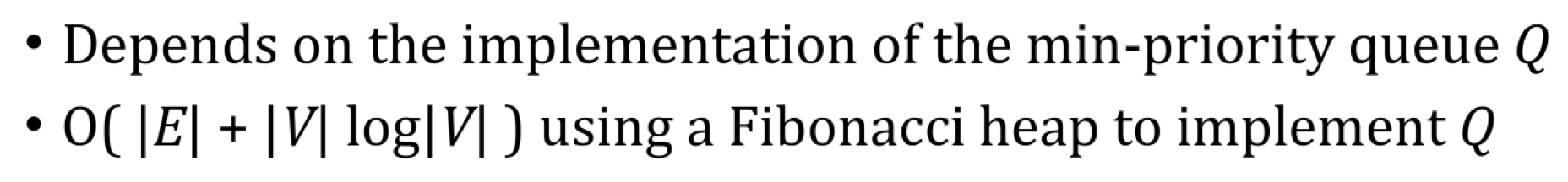# 数据结构与算法： 最小生成树

## Minimum Spanning Trees

Posted by R1NG on March 1, 2022 Viewed Times

# 最小生成树

## 1. 最小生成树的定义与最小生成树问题

### 1. 问题定义

1. $V$ 为需要连接的所有节点
2. $E$ 为所有可选的连通边组成的集合

$E = \{(u, v) ~ \vert ~ w(u, v) \neq \infty\}$
3. 对任何 $(u, v) \in E$, $w(u, v)$ 为连接 $u, v$ 的成本 (Cost).

$w(T) = \sum_{(u, v) \in T} w(u, v)$

$T$ 是 无圈的, 且由于它要将所有节点相连, 这意味着 $T$ 是 , 且 $G$ 为它的 扩展图 (Spanning Graph).

MST 问题就是: 求满足上述条件的 $T$.

### 2. 通用贪心算法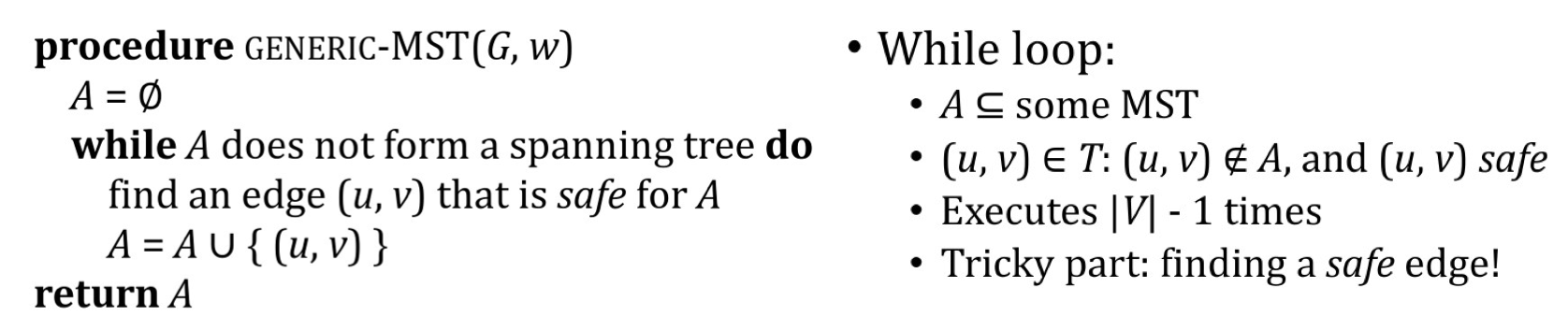1. $A \cup {(u, w)} \subseteq T$.
2. (u, v) 也是一条安全的边.

1. 在初始状态下, 第 $0$ 次循环执行前显然 $A$ 满足循环不变量的条件.
2. 在执行随后的任何一次循环时, 只有安全的边 (被某个 respect 最小生成子树 $A$ 的分割中穿过的 权重最小 的边) 被加入进 $A$ 中构成新的部分解.
3. 在循环终止时, 由于已知在任意一次循环中加入到 $A$ 中的边都属于某个 MST, 因此所得到的 $A$ 必然也是一个 MST.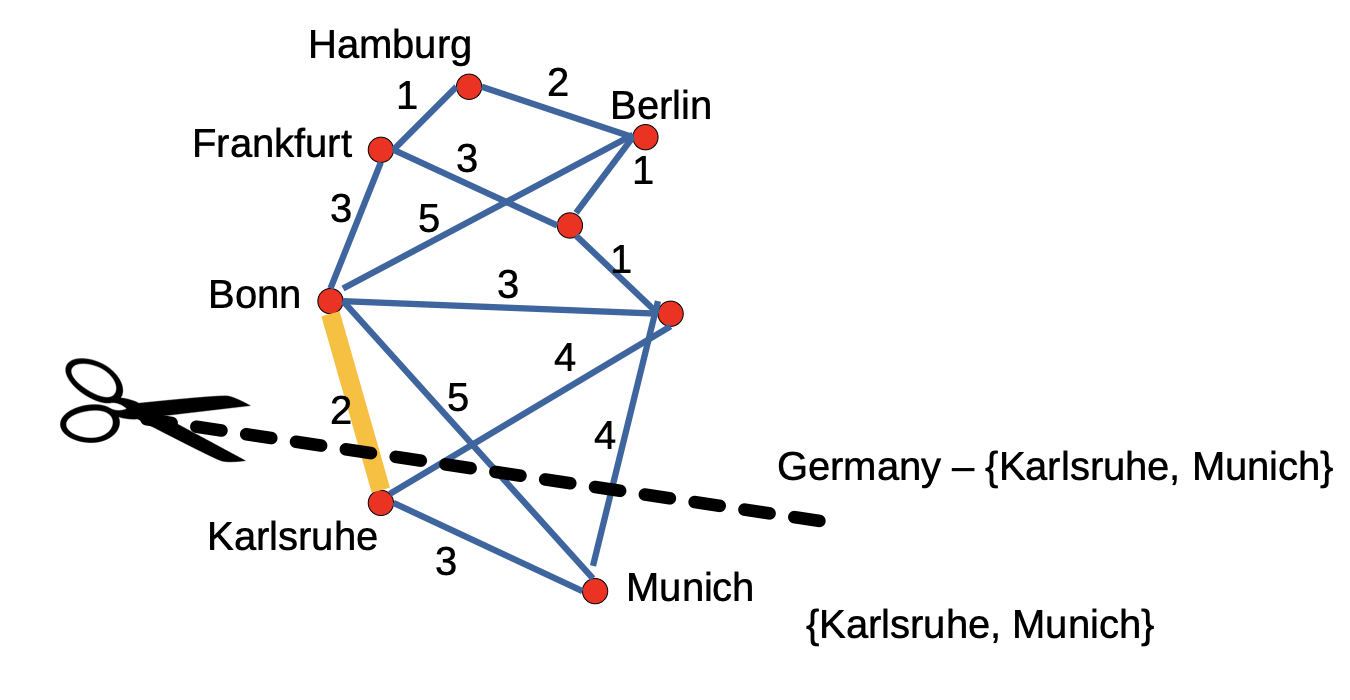1. 考虑无向图 $G = \langle V, E \rangle$, 且 $E =\lbrace(u, v) ~ \vert ~ w(u, v) \neq \infty \rbrace$.
2. 对图 $G$ 边集 $E$ 的子集 $A$, 它包含于某个图 $G$ 的 MST (最小生成树) 中.
3. 存在对图 $G$ 的分划 $(S, V-S)$, 且该分划对边集 $A$ 是 Respect 的 (不穿过 $A$ 中的任何一条边).
4. $(u,v)$ 是被分划 $(S, V-S)$ 所穿越的边中的最轻边.

## 2. Kruskal 算法

Kruskal 算法是基于上文所介绍的通用贪心算法的扩展. 和通用贪心算法不同的是, Kruskal 算法在每一步中都会优先选择 权重最小 的边, 用权值决定在构造生成树时先后加入的边的顺序. 其伪代码如下: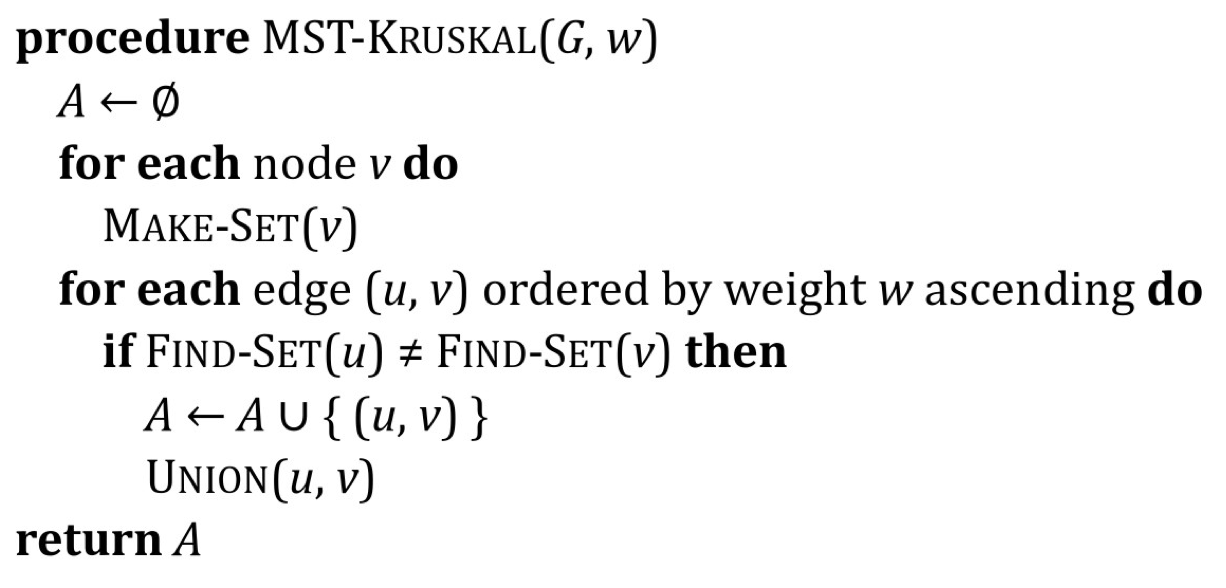1. Make-Set(x) 的作用是 创建一个只包含 $x$ (自然地, 也就以 $x$ 为代表) 的 并查集: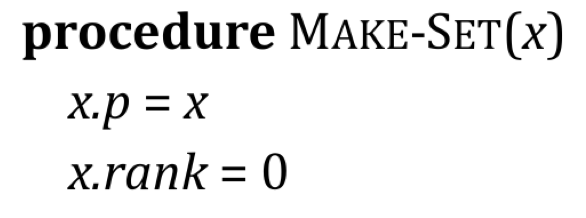且注意此处的 x.rank从 $x$ 所代表的并查集中的任意子节点到 $x$ 的最长路径所包含边数的上界 (Upper bound).

2. Find-Set(x) 所返回的是指向存有 $x$ 的集合的表示元 (Representative):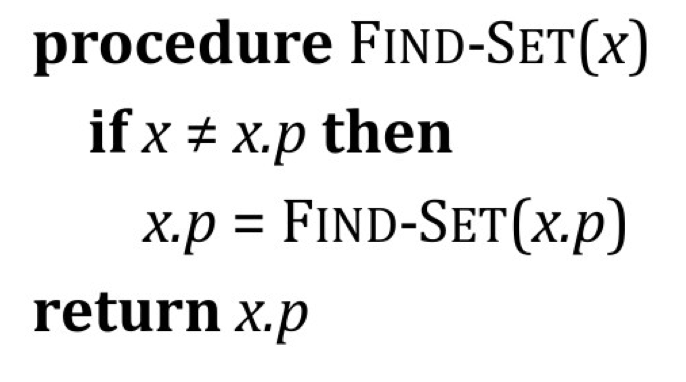3. Union(x, y) 表示将分别包含 $x$, $y$ 的两个不同的并查集合并, 合并规则为 Union By Rank.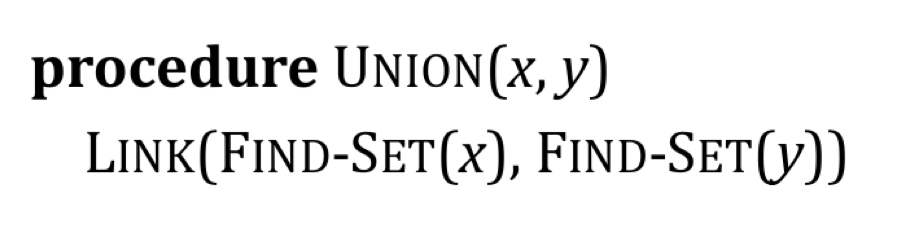Union By Rank 规则指: 在合并两个并查集时, 始终将 Rank 更小的那个合并到 Rank 更大的那个中, 且以 Rank 更大的并查集的代表元作为其自身的代表元.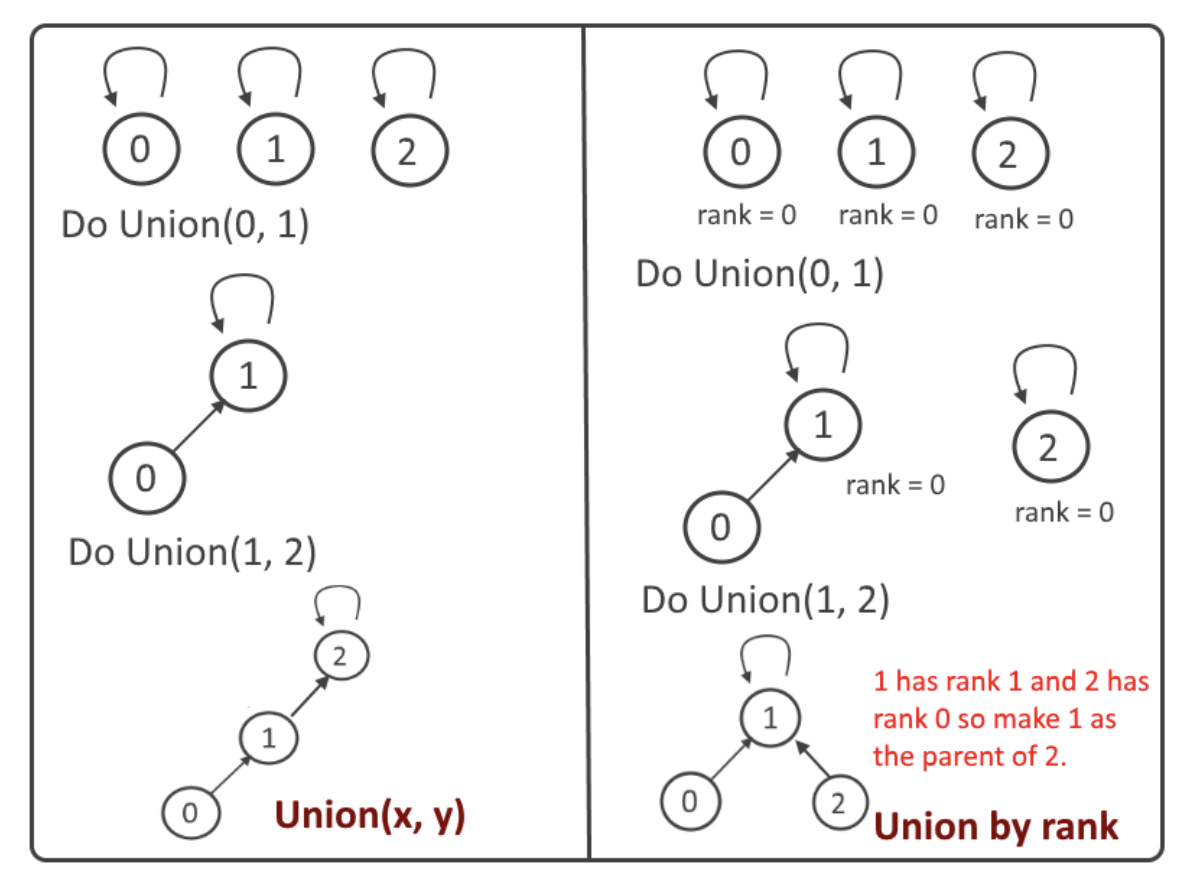$O(\vert E\vert \log(\vert V\vert)).$

## 3. Prims 算法

Prims 算法可视为基于 Dijkstra 算法基础上的扩展. 其基本思路是:

Prims 算法的伪代码如下: